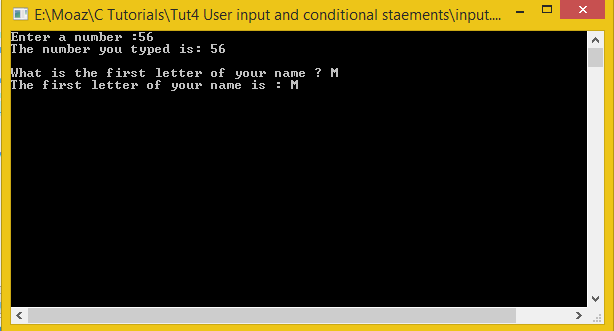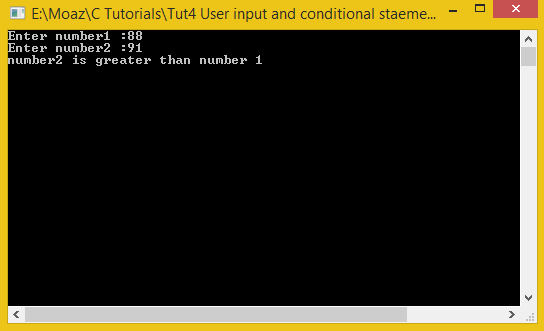# User input and Conditional statements

In this part you will learn: 1. Taking input from user 2. Conditional statements 3. C syntax 4. Showing output 5. Tabs and new lines In this tutorial I will teach you how take input from user and how to use conditional statements. Basic Step: Open Dev C++ then File > new > source file and start writing the code below.
`#include<stdio.h>#include<conio.h>`
These are the header files that we need for coding. The main header is stdio.h which provides us with most of the functions for coding in C and the second header files is just for using a function which can pause the screen until the user hits any key.
`int main(){	int number;	char alphabet;`
First of all we will declare an integer and an alphabet. In this program we will only use these variables to take input and we will simply print these variables just to check whether the program is taking correct input or not.
`printf("Enter a number :");	scanf("%d",&number); // scanf takes input remember ampersand sign &	printf("The number you typed is: %d ",number);	printf("\n\nWhat is the first letter of your name ? ");	scanf(" %c",&alphabet); // scanf takes input remember ampersand sign &	printf("The first letter of your name is : %c",alphabet);	getch();	return 0; // write now just write it as it is}//end main`
Now writing the print statements first and then we write the scanf function which is used to take the input from the user. In scanf function we first write the type of variable in which we are taking input by using the format specifier i.e incase of integer is %d and incases of character is %c and then we used the & symbol with our variable name this is to give compiler the address of the variable in memory , you will understand it later just write this & symbol as it is for now. In the end we just pause the screen using getch function and return 0 ( write it as it is for now ) and our program is complete. Execute > compile then Execute > run OutputUsing Conditional statements File > new source file The if , else and else if statements in c are called conditional statements. Now we will write a program to compare two numbers using conditional statements.
`#include<stdio.h>#include<conio.h>int main(){	int num1,num2; //declaring two numbers named num1 and num2	printf("Enter number1 :");	scanf("%d",&num1); // scanf takes input remember ampersand sign & 	printf("Enter number2 :");	scanf("%d",&num2); // scanf takes input remember ampersand sign &`
We will first write the header files and then start our main function. In the main function we declare two variables for number1 and number 2 and take user inputs in them. Then we start writing the conditional statements
`	if(num1 > num2) // if number1 is greater than number 2		printf("number1 is greater than number 2");	if(num1 < num2) // if number1 is less than number 2		printf("number2 is greater than number 1");		else   // 3rd possiblity number 1 is equal to number 2		printf("Both numbers are equal");	getch();	return 0; // write now just write it as it is}//end main`
There are three possibilities that a number is either greater, smaller or equal so we will write the conditional statements for all these possibilities. When number is greater, when it is smaller and if both possibilities are false then we go to else that both numbers will be equal then. After that we pause the screen and return. Execute > compile then Execute > run Output: